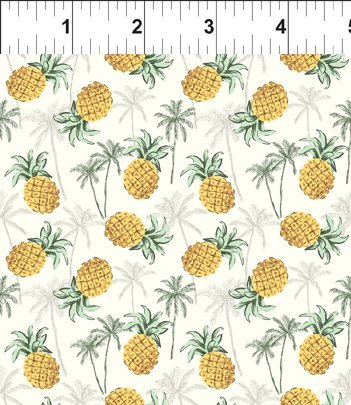# Mini Tropicals!

Tropical prints are always popular and when they are mini it's hard to resist! Can't decide? Get the fat quarter bundle with one of each!

 table div table+table+table+table div table{width:100%;padding:0}table div table+table+table+table div table img{width:96.23%;padding:0;float:none}table div table+table+table+table div table td{width:100%;padding:0 1.88% 18px}/* styles */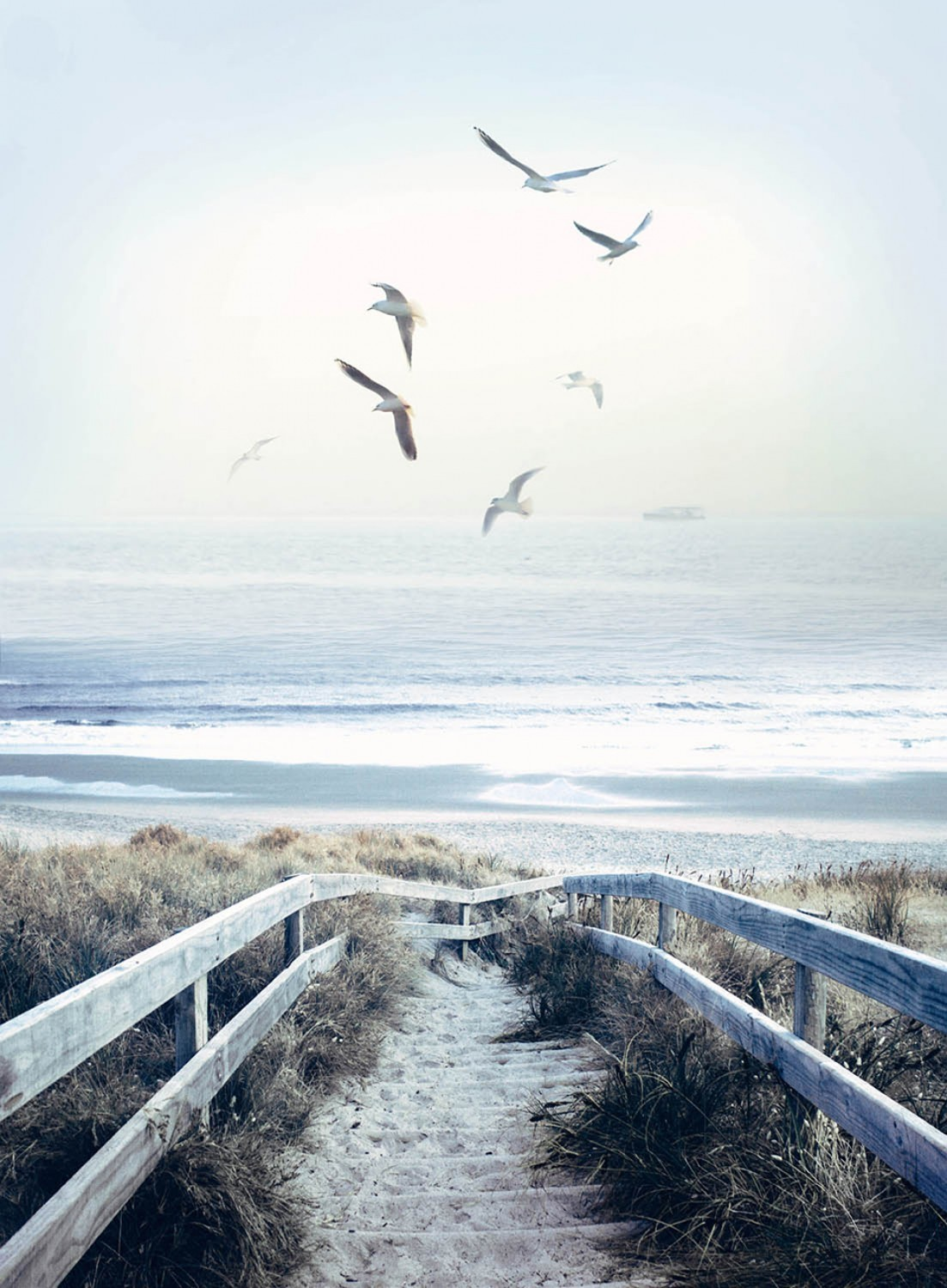New Fabrics!
Amazing Digital Prints from Hoffman - the crab panel is back and we also have this Atlantic Beach Boardwalk panel!
Sunflower Chalkboard Panel and coordinates have been very popular - don't wait!
Butterfly panel, Batiks and and awesome Atlas print that would make a great travel bag!

 table div table+table+table+table+table+table+table div table{width:100%;padding:0}table div table+table+table+table+table+table+table div table img{width:96.23%;padding:0;float:none}table div table+table+table+table+table+table+table div table td{width:100%;padding:0 1.88% 18px}/* styles */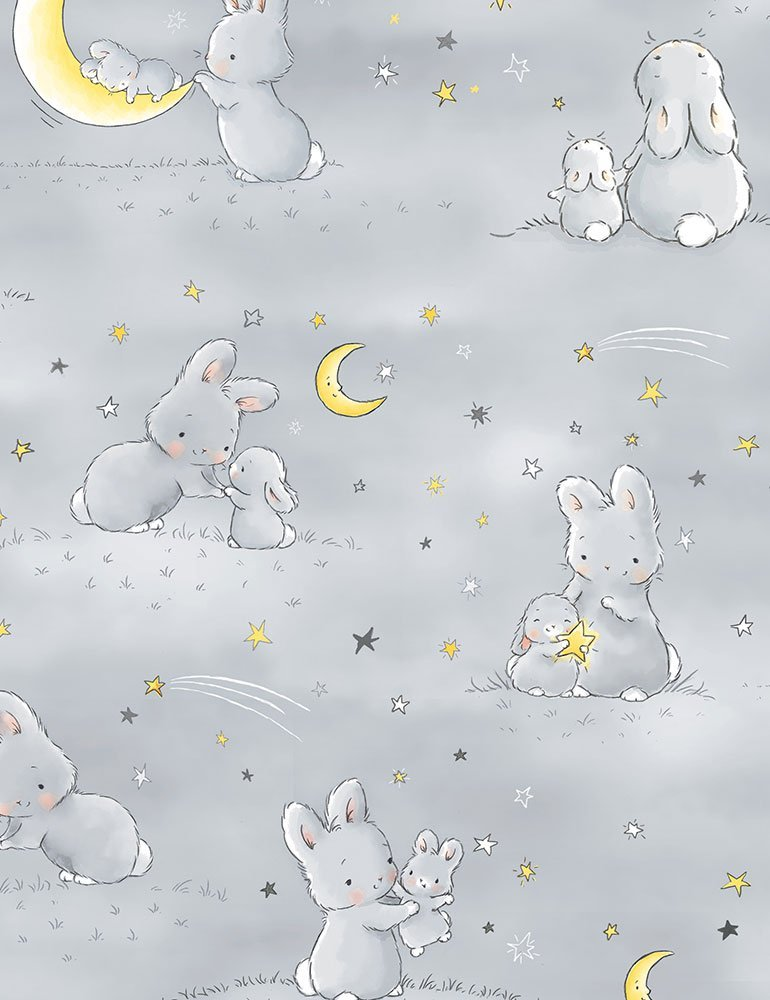# Little Star

Bunnies by the Bay adorable prints in the on-trend yellow and greys that all the new moms are asking for!

 table div table+table+table+table+table+table+table+table+table+table div table{width:100%;padding:0}table div table+table+table+table+table+table+table+table+table+table div table img{width:96.23%;padding:0;float:none}table div table+table+table+table+table+table+table+table+table+table div table td{width:100%;padding:0 1.88% 18px}/* styles */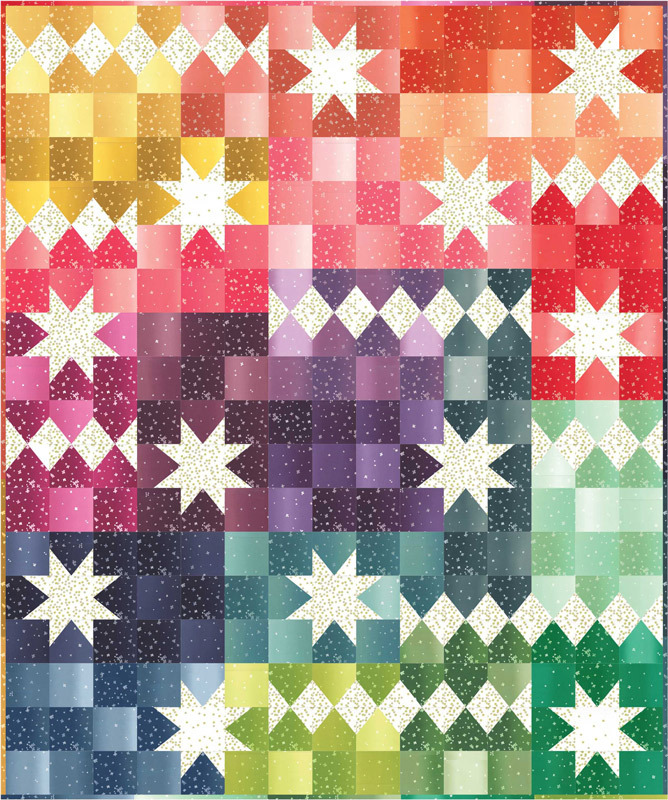Kits and Precuts!
2 new quilt kits, along with jelly rolls and charm packs - don't you just love it when all the fabrics are chosen for you?

 table div table+table+table+table+table+table+table+table+table+table+table+table+table div table{width:100%;padding:0}table div table+table+table+table+table+table+table+table+table+table+table+table+table div table img{width:96.23%;padding:0;float:none}table div table+table+table+table+table+table+table+table+table+table+table+table+table div table td{width:100%;padding:0 1.88% 18px}/* styles */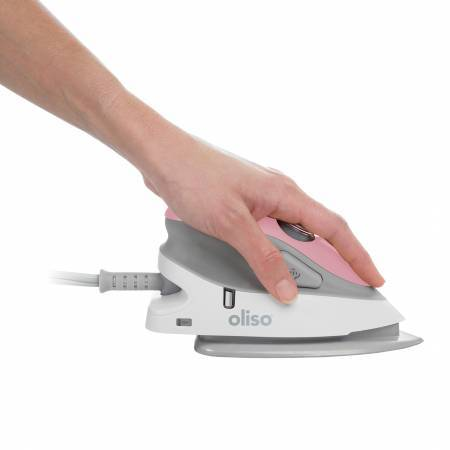# New Goodies!

The mini Oliso irons are here and everyone is loving them!
The Perfect 5 ruler is a handy, multi use ruler and the Perfect 5 Quilts book is, well, the perfect companion to it!
New patterns and an adorable pink bobbin case!

 table div table+table+table+table+table+table+table+table+table+table+table+table+table+table+table+table div table{width:100%;padding:0}table div table+table+table+table+table+table+table+table+table+table+table+table+table+table+table+table div table img{width:96.23%;padding:0;float:none}table div table+table+table+table+table+table+table+table+table+table+table+table+table+table+table+table div table td{width:100%;padding:0 1.88% 18px}/* styles */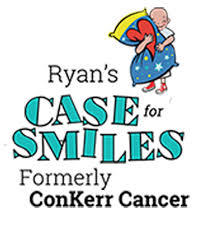# Pillowcase Sew Day!

Join us in the classroom on Thursday, November 14 from 10am to 2pm to sew pillowcases for Ryan's Case for Smiles!

 table div table+table+table+table+table+table+table+table+table+table+table+table+table+table+table+table+table+table+table div table{width:100%;padding:0}table div table+table+table+table+table+table+table+table+table+table+table+table+table+table+table+table+table+table+table div table img{width:96.23%;padding:0;float:none}table div table+table+table+table+table+table+table+table+table+table+table+table+table+table+table+table+table+table+table div table td{width:100%;padding:0 1.88% 18px}/* styles */# Next PMS Night is Friday, November 8

6pm to midnight
Treat yourself to a night of food, fabric and friends!
Who knows, you could be the next fat quarter winner!

## We need 4 more sign ups or we will have to cancel this month!

 table div table+table+table+table+table+table+table+table+table+table+table+table+table+table+table+table+table+table+table+table+table+table+table div table{width:100%;padding:0}table div table+table+table+table+table+table+table+table+table+table+table+table+table+table+table+table+table+table+table+table+table+table+table div table img{width:96.23%;padding:0;float:none}table div table+table+table+table+table+table+table+table+table+table+table+table+table+table+table+table+table+table+table+table+table+table+table div table td{width:100%;padding:0 1.88% 18px}/* styles */# Casual Sew 10am to 4pm

Friday November 8, 15, 22

 table div table+table+table+table+table+table+table+table+table+table+table+table+table+table+table+table+table+table+table+table+table+table+table+table+table div table{width:100%;padding:0}table div table+table+table+table+table+table+table+table+table+table+table+table+table+table+table+table+table+table+table+table+table+table+table+table+table div table img{width:96.23%;padding:0;float:none}table div table+table+table+table+table+table+table+table+table+table+table+table+table+table+table+table+table+table+table+table+table+table+table+table+table div table td{width:100%;padding:0 1.88% 18px}/* styles */# Upcoming Classes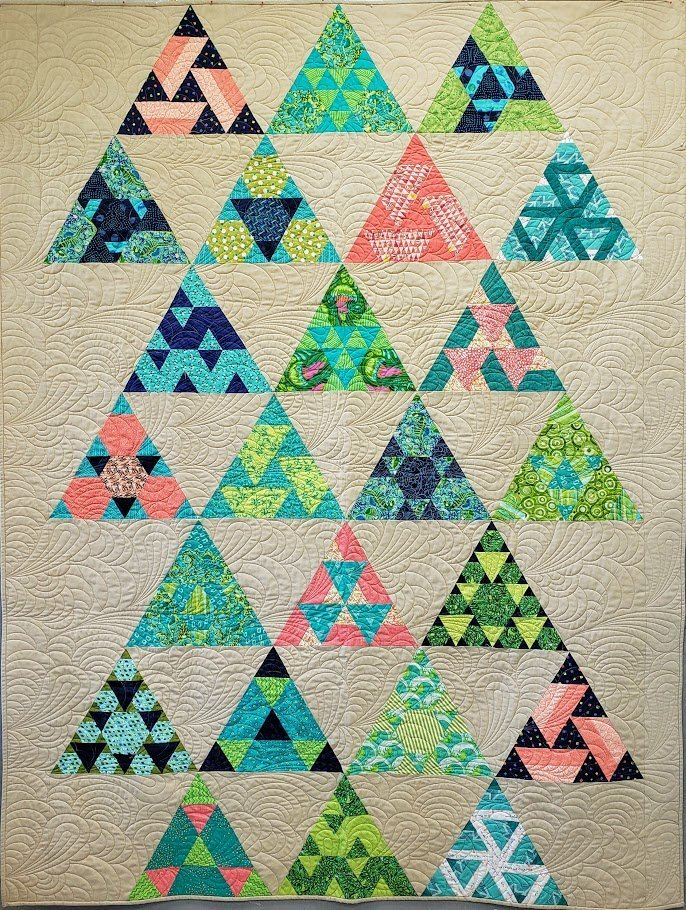# Toes in the Sand

So many different things you can do with the hexagon shape!
Class is Saturday, November 9 and 23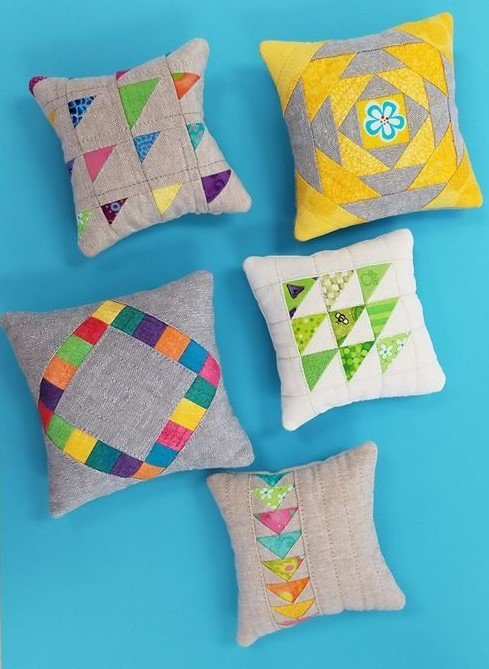# Plenty of Pincushions

Master tiny piecing and leave class with a finished pincushion (or two)!

Class is Sunday, November 10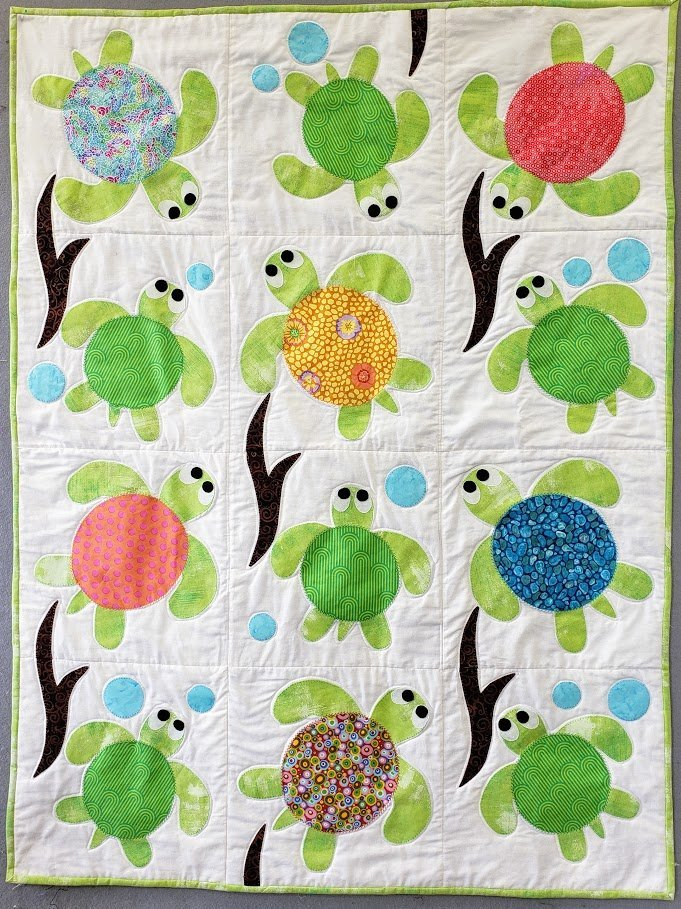# Sea Turtle Friends

Learn machine applique while making these adorable turtles!
class is Monday, November 18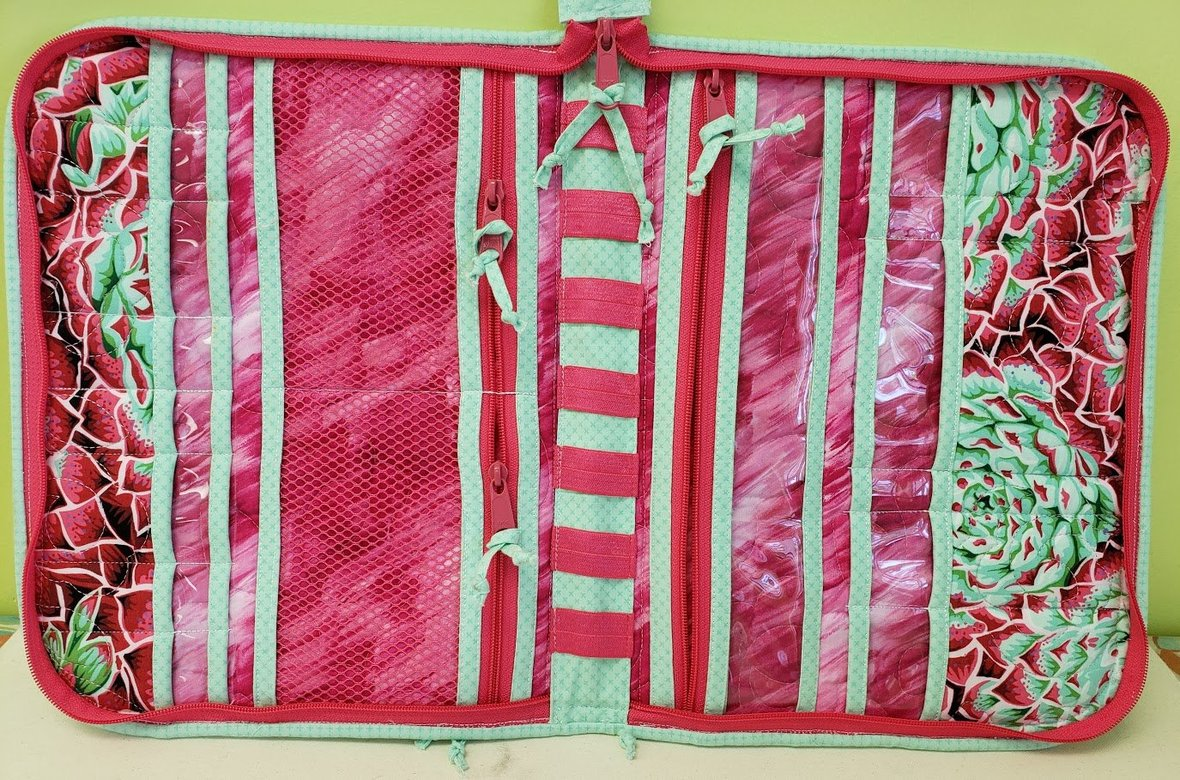# Running with Scissors

This zip-up tool case has so many amazing features - let Lanette guide you through the construction!
Class is 2 sessions, Wednesday, November 20 and December 4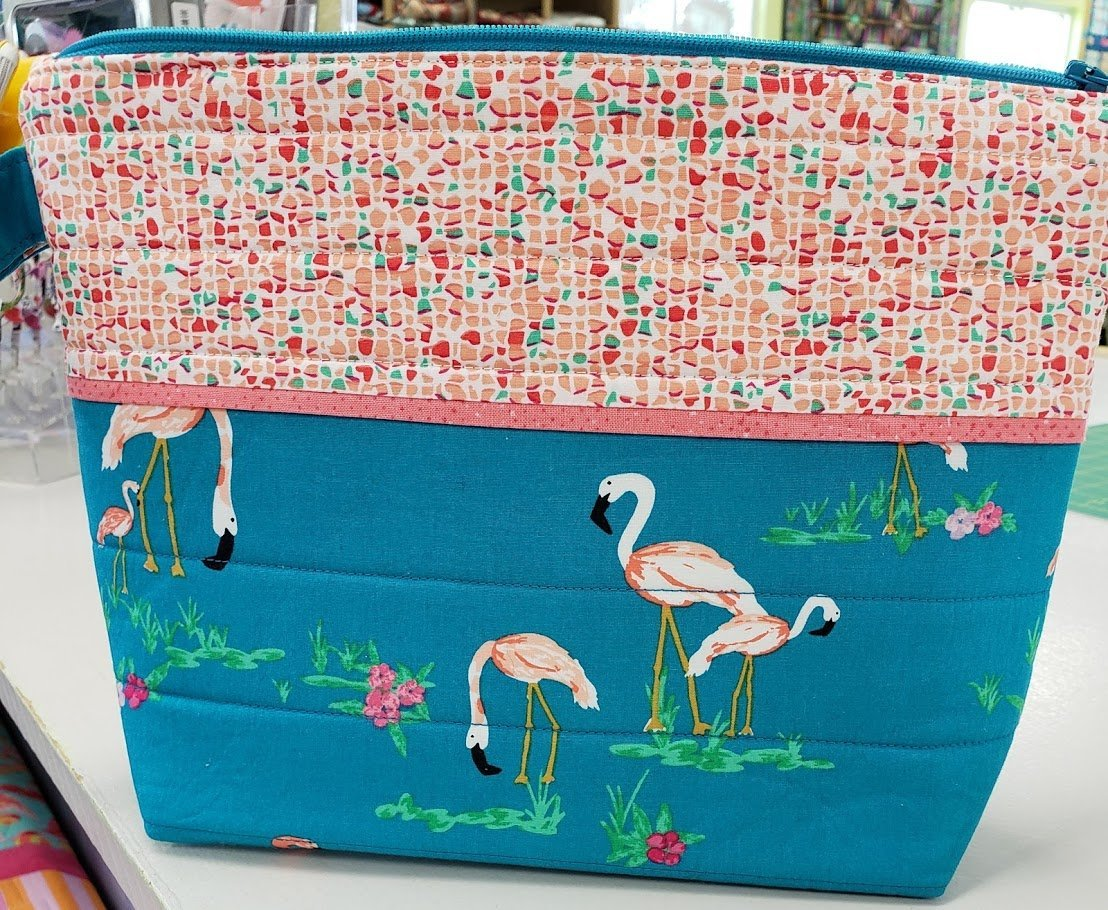# Sew Essentials Bag!

Margaret will show you how easy it is to put a zipper in a bag!
Class is Sunday, December 8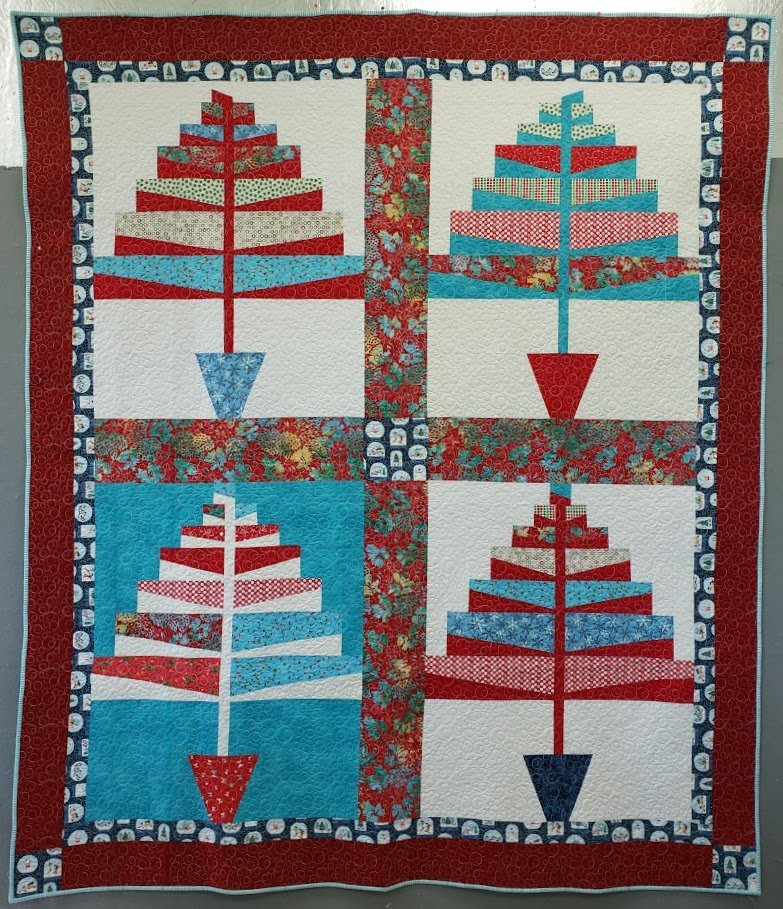# Christmas 1964

Whether you were there in 1964 or just like the vintage vibe, this is a fun quilt!
You could make just one tree for a wall hanging if you like.
Class is Saturday, December 14

 table div table+table+table+table+table+table+table+table+table+table+table+table+table+table+table+table+table+table+table+table+table+table+table+table+table+table+table+table+table+table+table+table+table+table+table div table{width:100%;padding:0}table div table+table+table+table+table+table+table+table+table+table+table+table+table+table+table+table+table+table+table+table+table+table+table+table+table+table+table+table+table+table+table+table+table+table+table div table img{width:96.23%;padding:0;float:none}table div table+table+table+table+table+table+table+table+table+table+table+table+table+table+table+table+table+table+table+table+table+table+table+table+table+table+table+table+table+table+table+table+table+table+table div table td{width:100%;padding:0 1.88% 18px}/* styles */# FB Live is the place to be Sunday at 7pm!

You will be the 1st to see new fabrics, notions, kits and other great things that you need to know about!

## We put together fun kits that often sell out that very night - you don't want to miss out!

There will be prizes, live demos and special pricing!

You will be able to purchase during the Live Broadcast and we can ship - sort of like Stuff Your Stocking but all in one night.
If you miss the FB Live - not to worry!

## You can view the replay on our FB Page AND you can still purchase at the special prices until 9pm Monday night!

We will go "live" at 6:55 and see what the hounds are up to, then
start the Soiree at 7pm (Eastern)

## Join me for a fun evening!

 table div table+table+table+table+table+table+table+table+table+table+table+table+table+table+table+table+table+table+table+table+table+table+table+table+table+table+table+table+table+table+table+table+table+table+table+table+table+table+table+table div table{width:100%;padding:0}table div table+table+table+table+table+table+table+table+table+table+table+table+table+table+table+table+table+table+table+table+table+table+table+table+table+table+table+table+table+table+table+table+table+table+table+table+table+table+table+table div table img{width:96.23%;padding:0;float:none}table div table+table+table+table+table+table+table+table+table+table+table+table+table+table+table+table+table+table+table+table+table+table+table+table+table+table+table+table+table+table+table+table+table+table+table+table+table+table+table+table div table td{width:100%;padding:0 1.88% 18px}/* styles */# Meet me in the Club House!

Cotton Patch Club House is a new FaceBook group and we want you to be a part of it!
You will find:
An online community for friends of Cotton Patch Quilt Shop!
A place to chat and share photos of your projects.
A place to ask quilty questions, share tips and demos
A place for you to find sneak peeks and early access to items on our FaceBook Live Sunday Soiree!

 table div table+table+table+table+table+table+table+table+table+table+table+table+table+table+table+table+table+table+table+table+table+table+table+table+table+table+table+table+table+table+table+table+table+table+table+table+table+table+table+table+table+table+table div table{width:100%;padding:0}table div table+table+table+table+table+table+table+table+table+table+table+table+table+table+table+table+table+table+table+table+table+table+table+table+table+table+table+table+table+table+table+table+table+table+table+table+table+table+table+table+table+table+table div table img{width:96.23%;padding:0;float:none}table div table+table+table+table+table+table+table+table+table+table+table+table+table+table+table+table+table+table+table+table+table+table+table+table+table+table+table+table+table+table+table+table+table+table+table+table+table+table+table+table+table+table+table div table td{width:100%;padding:0 1.88% 18px}/* styles */# Open 7 days a week for your convenience!

Monday through Saturday, 10am to 5pm
Sunday 12pm to 4pm

See you soon!

 table div table+table+table+table+table+table+table+table+table+table+table+table+table+table+table+table+table+table+table+table+table+table+table+table+table+table+table+table+table+table+table+table+table+table+table+table+table+table+table+table+table+table+table+table+table div table{width:100%;padding:0}table div table+table+table+table+table+table+table+table+table+table+table+table+table+table+table+table+table+table+table+table+table+table+table+table+table+table+table+table+table+table+table+table+table+table+table+table+table+table+table+table+table+table+table+table+table div table img{width:96.23%;padding:0;float:none}table div table+table+table+table+table+table+table+table+table+table+table+table+table+table+table+table+table+table+table+table+table+table+table+table+table+table+table+table+table+table+table+table+table+table+table+table+table+table+table+table+table+table+table+table+table div table td{width:100%;padding:0 1.88% 18px}/* styles *//* styles */ Freddie as a puppy in 2014. That look just says he has no idea how the toilet paper got there!
 table.module-46{width:89.25%;padding:0}table div table+table+table+table+table+table+table+table+table+table+table+table+table+table+table+table+table+table+table+table+table+table+table+table+table+table+table+table+table+table+table+table+table+table+table+table+table+table+table+table+table+table+table+table+table+table+table div table{width:89.25%;float:none;margin-left:auto;margin-right:auto;padding:0}table div table+table+table+table+table+table+table+table+table+table+table+table+table+table+table+table+table+table+table+table+table+table+table+table+table+table+table+table+table+table+table+table+table+table+table+table+table+table+table+table+table+table+table+table+table+table+table div table a{border:0 none;text-decoration:none}table div table+table+table+table+table+table+table+table+table+table+table+table+table+table+table+table+table+table+table+table+table+table+table+table+table+table+table+table+table+table+table+table+table+table+table+table+table+table+table+table+table+table+table+table+table+table+table div table img{width:100%!important;border:0 none;text-decoration:none}table div table+table+table+table+table+table+table+table+table+table+table+table+table+table+table+table+table+table+table+table+table+table+table+table+table+table+table+table+table+table+table+table+table+table+table+table+table+table+table+table+table+table+table+table+table+table+table div table td{width:100%;padding:0}/* styles */
 Like   Pin   +1